## 8.15.2012

### Decision of unsolvable equations

In comments to the article infant "Wunderkindes and cretin with blondes" (in Russian language) me offered to decide a few equations it is "known from a digit, that a decision is not present". These equations look like the following:

1) x + 2 = x

2) √x = -1

3) x/0 = x

Probably, on mathematicians these unsolvable equations operate, as a boa on rabbit and infuse with in their souls the awesome trembling. I am deprived such superstitions. Moreover, the author of this idea, Vag, tossed up to me a magic stick:

"Decisions does not have" means that it is WELL-PROVEN that such circumstances at that problem abode by ARE not.

Today we will consider circumstances at that terms at least one of equations observed. Consequently, at least one unsolvable equation we will decide now.

But to begin would I like not from a decision, and from an answer to the question: "Where these unsolvable equations appeared from?" Me it seems to, here from where...

...A mother bought to the child a new toy are blocks with an alphabet. She collected a few words from blocks, read them and gave blocks to the child. A mother took up the everyday businesses.A child with enthusiasm began to fold blocks. Finishing to lay out series from the blocks taken by chance, he put question to the mother:

- That is here written?

- Nothing. You laid down letters wrong, - a mother explained, - When will learn letters, then you will be able correctly to fold blocks.

A child was very offended and burst into tears. What a more mother tried to quiet him, the he cried stronger. Then a mother said:

- Well, try once again, I necessarily will read you.

Bustling a nose, a child at random took a few blocks and laid down them in series.

- What did I write? - he asked with tears.

Farther not to disorder a child, a mother answered:

- In mathematics a certain integral is so designated in limits from Old Testament to nine evening, taken on the surface of asynchronous point.

A child quieted down puzzled. He understood nothing, but something disturbed him in this phrase. A bit thinking, he asked:

- And will a fairy-tale be at nine o'clock of evening?

- It will be necessarily. Where will she go? - a mother answered very confidently.

Very attentively looking to the mother in eyes, a child by touch took a few blocks and laid down another abracadabra.

- And it what did I write? - he asked with distrust, not tearing away a look from mother eyes.

If a mother will not look at blocks will say that is written there, she means cheats him. If will look - she means that is written there really reads.

A mother looked another portion of incoherent words at blocks and crfpfkf. A child was happy. He has the most clever mother! Farther this game proceeded until child not tired of. He really had a clever mother that knew many clever words.

A child grew up then, learned letters, began independently to fold words from blocks. When he became adult, he forgot playing blocks, but faith that for him the most clever mother, remained...

Just as a child made blocks, mathematicians worked out unsolvable equations from mathematical symbols. A child grew and became adult, mathematicians so remained in pampers of the determinations.

Now we will pass to business.

Decision of the first unsolvable equation

x + 2 = x

This equation traditionally can be taken to next equality

2 = 0

As this equality did not look wildly, but in mathematics such quite possible. I will say anymore, the first equation is only one equation from the system of two equations, that has one common decision. The second equation looks so:

2 + х = 2

This equation is usually taken to equality

х = 0

The decision of this system of equations looks so:

х ⊥ 2

It means that the numbers taken by you are on perpendicular numerical axes, therefore the rules of ordinary arithmetic give such result.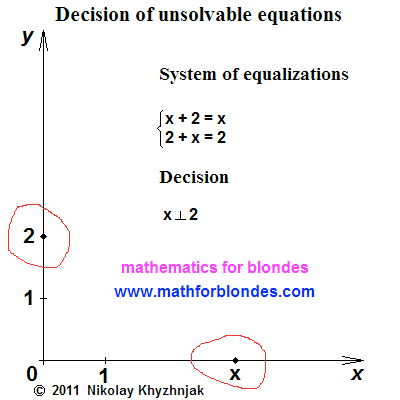I especially want to underline that all the known rule "from transposition of elements a sum does not change" in this case stops to work. A result depends on that, what number you take for basis at implementation of action of addition.

The further decision of this system of equalizations is possible two methods resulting in different results.

First method. Turn of one of numerical axes on 90 degrees and passing to the rules of ordinary addition. Undeniable equality will ensue:

x + 2 = x + 2

n this case the laws of symmetry of mathematical actions begin to work.

Second method. Remaining in the rectangular system of coordinates, to apply the methods of vectorial algebra and find a sum on the theorem of Pythagoras, where х and 2 are the cathetuses of rectangular triangle, and result of addition - by a hypotenuse. If you consider that application of facilities of vectorial algebra is impossible, when on the ends of sticks the tips of pointers are absent, then it is your personal problems already. In this case both equalizations of the system are taken to the identical decision:

x + 2 = √(x² + 2²)

It is done away with the decision of this unsolvable equalization. you need to be only determined with the desires - what result needs you.

Decision of the second unsolvable equalization.

√x = -1

This decision caused most difficulties from the simplicity. We erect both parts of equalization in a square and get an answer:

x = 1

This equalization is taken to equality:

+1 = -1

In the parallel instances of mathematics usually begin to dissert upon the modules of numbers. I will say that before to put signs before numbers, it is needed to understand sense of positive and negative numbers.

Decision of the third unsolvable equalization

x/0 = x

The decision of this equalization is taken to equality:

1 = 0

Personally for me there is nothing unusual in this equality. This one of basic equalities of mathematics, without that mathematics in principle is impossible. If our mathematicians until now do without this equality, then only due to the pampers. At exposition of mathematics on the pages of this web-site I will repeatedly call to this equality.

To accept the solutions offered by me or not accept is this your personal file. Monkeys too nobody compelled to go down on earth. Many of them until now on branches bruise along and fully happy without pampers))))

### Theorem about parallel lines, thought of in motion

While I wrote about the types of triangles, I was visited by one idea - I thought of a theorem about parallel lines. She sounds so:

distance between parallel lines (by planes, volumes etc.) it is impossible to define mathematical methods

To engage in proof of this theorem about parallel lines we will not be now with you. We will leave proof on the future. If are wishing to prove or refute this theorem - occupy.

You can argue that there are very much tasks in the textbooks of mathematics, where it is needed to find distance between parallel lines and all of them decide mathematical methods. I agree, but... In the textbooks of mathematics I am much such, what is not present in mathematics. Yet more in mathematics what is not present in the textbooks of mathematics. Little by little we will understand with you, who is who in mathematics and where it undertakes from. And we will begin from unsolvable equalizations.

## 7.08.2012

### What trigonometric circle?

What trigonometric circle? Yet he is named trigonometric circumference. Question interesting enough. We will not go into the details of distinctions of concepts "circle" and "circumference". Although, for blondes I will explain that a circle is a coin or hole from a bagel, a circumference is this wedding ring or bagel. Coming from classic determination of trigonometric functions, more correct to talk "trigonometric circumference", as the question is about the set of points and their coordinates, but not about a flat figure and her area. But in the Internet much more often search a "trigonometric circle" exactly, probably because collecting so less letters is necessary.

Before we will pass to formulation of determinations of trigonometric functions with the use of portrait of tangent, we need to understand, what a trigonometric circle differs from all other circles. In reasoning we will use one mathematical focus sense of that is based exactly on these differences.

We will take the picture of circumference from determination of trigonometric functions. He looks here so.On a picture the ordinary circumference of arbitrary radius is represented. As in the center of circumference cheekily fell to pieces the cartesian coordinate system, then all points of this circumference have vertical and horizontal coordinates. Radius of circumference and coordinate of points expressed by numbers. The intersections of axes of coordinates and circumference are determined by the size of radius of circumference and numeral him equal to.

In an order to convert an ordinary circumference into trigonometric one, it is needed to divide all elements of circumference into the radius of circumference. Letters of "X" and "Y" are the tags of the system of coordinates, designating her obtrusive presence on our picture. These letters by a radius are not divided, as well as letter of "B", designating a point on a circumference. Point "0"(zero) as a result of dividing by a radius so will remain a point "0"(remember, the zero divided by any number equals a zero?). Corner alpha it is possible to divide into a number, but it is impossible to divide into a radius circumferences(by the way, here to you the prepared proof that a division does not make sense) :))), therefore a corner it is better not to touch, in order to avoid large troubles(from all present person on a picture, a corner is the steepest element, he is in all trigonometric functions in the natural kind). For us remain only radius of circumference and coordinate points of "B", that we can divide into the size of radius of circumference.

Clear that at dividing of radius of circumference by an exactly such radius, we will get unit as a result. And at dividing of coordinates of point of circumference by a radius we will get the values of sine and cosine of corner alpha(on determination of trigonometric functions). That is our transformations look on a picture.Now we need only to substitute all radiuses by units and coordinates of point on a sine and cosine of corner alpha and a trigonometric circumference will turn out for us. We even can set forth determination of single circumference (let child prodigy teach):

a circumference with a radius equal to unit is named a trigonometric circumference, if there is a center of the cartesian system of coordinates in the center of circumference.

This determination far not complete and does not take into account many nuances on that mathematicians take no notice usually. But about it we better will talk at the study of properties of trigonometric functions. And while we will look at a picture, as a trigonometric circumference looks in all beauty.Actually, this is a trigonometric circle. If on him to place the values of different corners and corresponding to them values of sine and cosine, then we will get the trigonometric circle of sine and cosine.

Now about that focus that we will do during formulation of determinations of trigonometric functions. Here we substituted the radius of circumference by unit, and there we will act vice versa - we will replace unit the radius of circumference. Id est, from a trigonometric circumference to pass to the circumference of arbitrary radius, as that is required by a textbook on mathematics.

### Trigonometric functions determination through a triangle

Trigonometric functions can be determined on a rectangular triangle. On a picture you can see determinations of all trigonometric functions through the elements of rectangular triangle.It is another icon for hanging out on a wall and overlearnings of text. We this we will not engage in. We will look better, as possible to combine a rectangular triangle and circumference. For this purpose we will combine two pictures: circumference from classic determination of trigonometric functions and our triangle. We will place a triangle next to a circumference. That is it looks.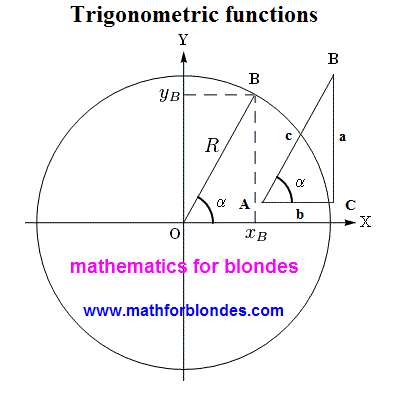As see, pictures are practically identical, and component elements are named differently in them. Identically mark only corner alpha and point of "B". Now we will impose a triangle straight on a circumference. All graphic images accepted for a circumference we will save, and a rectangular triangle we will underline red lines on the inside (type we will lead around the contour of triangle lipstick).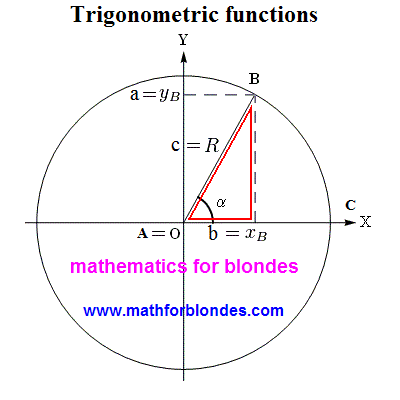As be obvious from a picture, the hypotenuse of rectangular triangle grows into the radius of circumference, cathetuses become equal to the coordinates of point. It as in a human language - a the same concept in different languages is designated by different words. This distinction in a pronunciation does not give to us to understand foreign languages. Approximately the same takes place in mathematics. Some consider determination of trigonometric functions on a rectangular triangle primitive. If you want to understand mathematics, will memorize the following: there are not primitive things in mathematics. There are primitive creatures counting itself very clever. Exactly desire to seem more clever than other, resulted in that mathematicians almost nothing is understood in mathematics.

Specially I will quote a phrase from Russian-language Wikipedia, where talked about determination of trigonometric functions on a triangle: "This determination has some pedagogical advantage, because does not require introductions of concept of the system of coordinates, but also such large defect, that it is impossible to define trigonometric functions even for obtuse angles that must be known at the decision of elementary tasks about amblygons". Honestly speaking, like thick ignorance of mathematicians simply shocks me. What triangle are trigonometric functions determined for? Correctly, for RECTANGULAR. Let though one mathematician will show to me RECTANGULAR TRIANGLE With OBTUSE ANGLE. I swear to you, as soon as I will see this geometrical miracle, I here will invert the portrait of tangent heels over head and I will hang oneself on a cosine. Not a single mathematician understands really, that the decision of "elementary tasks about amblygons" through trigonometric functions is ALWAYS taken to breaking up of one amblygon on two rectangular triangles?

Now I will show the most primitive example of existence of trigonometry you in life. More primitive, probably, is not already.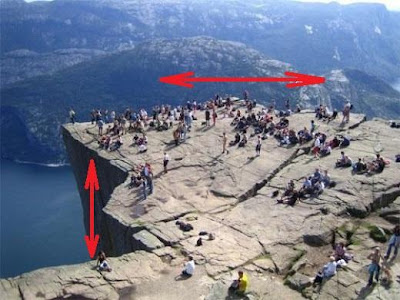Look at a photo - how many human bodies serenely stationed oneself on the horizontal plane of rock, at the same time there is nobody on a vertical plane. Why? And because trigonometric functions for perpendicular direction have quite another values. For us with you basic sense of trigonometric functions is that exactly trigonometric functions determine our possibilities.

## 7.03.2012

### Trigonometric functions are determination

Determination of trigonometric functions I took from Russian-language part of Wikipedia and designed as a picture. You can look and read.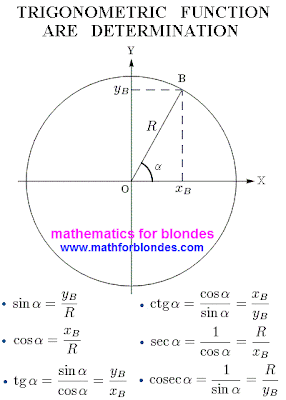Picture, naturally, I a bit did. In Wikipedia text of determination of trigonometric functions is written about one - about the coordinates of point and radius of circumference, and on a picture quite another is drawn. Probably, an artist from Wikipedia looked not in the that page of textbook on mathematics. Well yet, that he the textbook of mathematics with a textbook on biology did not entangle. Here that turned out for him.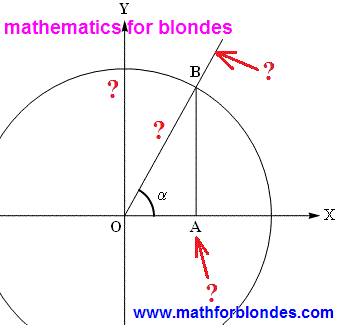Many of you can say: "I do not understand trigonometry. That to do"? Do the same, that do all mathematicians - bluntly teach determinations and, if necessary, pronounce them aloud, as talking parrots. For this purpose unseal my picture, hang up it above a bed and every evening before a dream read determination of trigonometric functions instead of prayer. I will open to you one great secret of mathematicians : those, who rewrites the determination given above from a textbook in a textbook, very small understand in mathematics in general, and quite nothing is understood in trigonometric functions in particular. Those, who understands, what trigonometric functions and able to use them, on all Universe travel in flying piattis. We hardly tear away the priests from a terrene through airplanes and rockets. By the way, would you like to exchange it stinking petrol car on an elegant flying object of UFO type?

If did you already read my notes How to memorize trigonometric functions? and the Trigonometric functions, then to you teaching nothing is not necessary. All, that it is needed for formulation of any determination of trigonometric functions, you know already. Later I will show you, as to use these knowledge. And while we will keep reading Wikipedia, where talked about determination of trigonometric functions for a rectangular triangle.

## 7.02.2012

### Trigonometric functions

Trigonometric functions is simply. At the last time we considered the portrait of tangent, today we will expose the secrets of practical application of this masterpiece of painting.

Any trigonometric function is the result of division. It needs to be memorized forever. We divide something by something and as a result get a trigonometric function. That on what divided in trigonometric functions? Variants are different here. I suggest not to learn by rote you on memory all, in respect of trigonometric functions. Let us try simply to apply the portrait of tangent and minimum set of necessary knowledge.

And so, a tangent is a sine on a cosine. As we said hardly higher, in a tangent a sine is divided by a cosine. A division in mathematics can mark a fractional line or two points. We will transform our portrait of tangent in the record of partaking with two points. Here that turned out for us.Most important, what we are taught by this picture, so it to those places, where it is needed to search sines and cosines. In the system of denotations accepted by mathematicians, sines always are situated on a vertical line - it upwards-downward. Cosines always are situated on a horizontal - it to the left-right (or aside). That is a complete set will look from 6 trigonometric functions in our variant of denotations. For evidentness a sine and cosine are depicted by a different color and we will add fractional hyphens wherein without them not to do.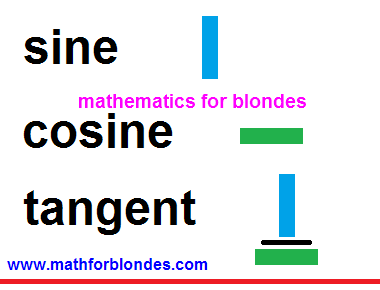Do not be frightened such amount of pictures. That it is represented in overhead part, above a red line, we almost learned already. We will repeat:

## a sine - it upwardsa cosine - is asidea tangent - it to divide a sine into a cosine

Do you see? The half of trigonometric functions we learned already. With the second half, that under a red line, yet simpler - invert that you learned already heels over head. Remaining trigonometric functions are shots reverse already known to us. We swap a numerator and denominator - all is ready already for us.

With a cotangent all is simple. A cotangent is this attitude of cosine toward a sine. With gimbleting of tangent no problems arise up - he has both a numerator and denominator. But as to be with a sine and cosine? We do not have denominators of these shots in fact! Do not worry, any number has a denominator that in mathematics, writing is not accepted is unit. In a secant and cosecant exactly this unit appears in a numerator, and a cosine and sine are written in a denominator.

It is important to memorize! In the pairs of the names of trigonometric functions a secant is a cosine and cosecant - a sine can be only one prefix to on two names. If we take a sine for basis, then a reverse shot will be named cosecant. If we take a cosine, then a reverse shot is named secant, because one prefix to we already used up on a cosine.

It is important to memorize! In the pairs of the names of trigonometric functions a secant - is a cosine and cosecant - a sine can be only one prefix co on two names. If we take a sine for basis, then a reverse shot will be named cosecant. If we take a cosine, then a reverse shot is named secant, because one prefix co we already used up on a cosine.

In principle, it fully sufficiently, in determinations of trigonometric functions to feel, close as an oyster in water. A question remains not found out, that at a sine and cosine to write in a denominator instead of unit. In fact we said in beginning, that trigonometric functions are a result of division(relation) or shot. Do not worry, mathematicians will prompt us, that exactly they want there to see. For this purpose there are determinations of trigonometric functions. We will talk next time about them.

### Why is a zero not a number?

Why is a zero not a number? Let us conduct a scientific experiment. We will take any number and will execute the simplest mathematical action - addition or deduction.

Simply so to execute a mathematical action will not turn out. One number and one sign of mathematical action are simply two mathematical symbols, standing alongside. Single character designates a number, the second symbol designates an action.

7 +
7 –

In order that an action happened, it is needed yet something. Only then we will get a result. And this result depends on that we take exactly. If we will take other any number, then the first number will change. The result of this change we write down after equal sign.

7 + 2 = 9
7 – 2 = 5

number + number = result
number - number = result

And that will be, if we will take a not number? For example, will we add salt, will add a paint, will decrease a temperature? That will be with a number, if to salt him? Nothing. A number will remain unchanging.

7 + salt = 7

Now we will try to paint a number.

7 + paint = 7

A number did not change again. But as regarding that, to push in our number in a refrigerator and lower his temperature?

7 - temperature = 7

Why does take place so? Because salt, paint, temperature numbers it is not been. A number and not number inter se do not co-operate. If to take them and make an effort with them to do anything, for us nothing will turn out. What number we took at the beginning, the same we get in the end.

number + not number = number and not number
number - not number = number and not number

Mathematicians and philosophers will argue that a number is an abstract concept that does not exist in the wild, and salt, paint, temperature, is the real things. Exactly from a difference abstract and real nothing takes place. The abstract can not influence on the real, real can not influence on abstract. What, I fully agree with the conclusions of mathematicians and philosophers. They are absolutely right - for a change real the real is needed, for a change abstract the abstract is needed.

In the second part of our experiment we will try to the abstract number to add anything abstract. Well, for example, soul. In hands this thing nobody held, she is not fixed scientific devices. The soul is this cleanly abstract concept. We will look at a result.

7 + the soul = 7
7 - the soul = 7

As see, even many abstract concepts are not able to run the number, because not all abstract concepts are numbers.

Here time to conduct an experiment with a zero, the same abstract concept, as well as number came now. We will add and will take away a zero from a number and will look at a result.

7 + 0 = 7
7 – 0 = 7

As a result of addition or deduction of zero a number remains unchanging. And takes place so because zero, as well as all other not numbers, a number is not. A zero does not possess all those properties that is possessed by numbers.

For plenitude of the conducted experiment it is needed to consider the mathematical operations of increase and division. Multiplying and dividing by a zero in mathematics has a result different from the originally taken number. But let us remember history of development of mathematics.

Addition and deduction appeared at first, an increase and division appeared then. Probably, somewhere a zero appeared after it. There was it in those times, when a faith in God was basis of existence of man. About no results of experiments there could not be speech, if these results conflicted with a faith. Here on this faith and the results of increase and dividing are founded by a zero. Someone said that a zero was a number and in it believed. Someone offered the result of multiplying by a zero - believed in a result. In him we are pin faiths until now: any number increased on a zero equals a zero.

With dividing by a zero there were problems. Nobody was able to offer nothing clever, such, that all believed in it. Mathematical experiments with the results of dividing by a zero give contradictory results and trench upon bases of mathematics. A compromise variant was therefore found is a phrase, "dividing by a zero is impossible". It did not mix to believe that a zero is a number, and did not result in the necessity of revision of other substantive provisions of mathematics. Absence of result of division of number on a zero confirms circumstance that in this case we deal with a not number.

Conclusion: ZERO IS NOT NUMBER